# Lines And Angles Solution of  TS & AP Board Class 9 Mathematics

###### Exercise 4.1

Question 1.

In the given figure, name:

i. any six points

ii. any five line segments

iii. any four rays

iv. any four lines

v. any four collinear points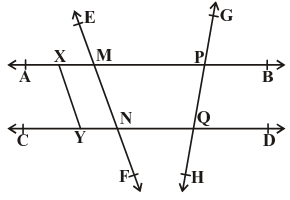i) A point specifies the exact location and looks like a small dot.

∴ The points in the given figure are A,B,C,D,E,F,G,H,M,N,P,Q,X,Y.

ii) A part of a line with two end points is a line segment.

∴ The line segment in the given figure are AX,XM,MP,AM,AP,AB,XB,XY…etc

iii) A ray has a starting point but no end point. it may go to infinity.

∴ The ray in the given figure are A,B,C,D,E,F,G,H.

iv) A line goes without end in both direction.

∴ The line segment in the given figure are AB,CD,EF,GH.

v) if three or more points lie on the same line, they are called collinear points

∴ The collinear points in the given figure are AXM,EMN,GPQ,CYN…

Question 2.

Observe the following figures and identify the type of angles in them.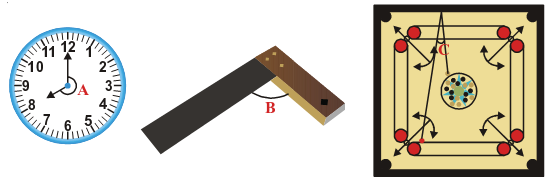A) The given figure shows the larger angle area, so it is reflex angle. The angle is more than 180° and less than 360°.

B) The given figure shows the right angle that is B = 90°

C) The given figure shows the smaller angle area, so it is acute angle. The angle is more than 0° and less than 90° .

Question 3.

State whether the following statements are true or false :

i. A ray has no end point.

ii. Line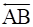is the same as line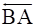.

iii. A ray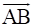is same as the ray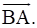iv. A line has a define length.

v. A plane has length and breadth but no thickness.

vi. Two distinct points always determine a unique line.

vii. Two lines may intersect in two points.

viii. Two intersecting lines cannot both be parallel to the same line.

(i) False

A ray has a starting point but no end point but it goes to infinity.

(ii) True

A line goes without end in both direction. so,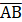is same as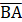(iii) False

A ray has a starting point but no end point but it goes to infinity. so

a rayis not same as(iv) False

A line goes without end in both direction. so, it does not have a define length.

(v) True

A line goes without end in both direction. so,is same as(vi) True

If two points are joined together then forms a line.

(vi) False

Two lines intersected at one point.

(vii) True

A parallel line does not have any intersecting point.

Question 4.

What is the angle between two hands of a clock when the time in the clock is

(a) 9’O clock

(b) 6’O clock

(c) 7:00 PM

(a) Let draw the 9’O clock and find the angle between the lines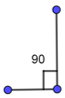∴ The angle is 90°

(b) Let draw the 6’O clock and find the angle between the lines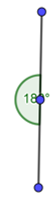∴ The angle is 180°

(c) Let draw the 7.00 PM and find the angle between the lines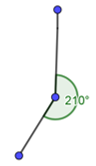∴ The angle is 210°

###### Exercise 4.2

Question 1.

In the given figure three lines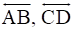and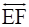intersecting at O. Find the values of x, y and z it is being given that x : y : z = 2 : 3 : 5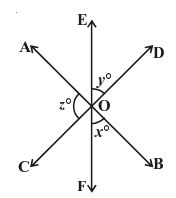From the given, the three angles are x,y,z

If the two lines intersect at a point then its vertically opposite angles are equal.

∴ A = x, B = z and C = y

We know that,The sum of all the angles around at a point is equal to 360°

∴ A + B + C + x + y + z = 360°

⇒ x + y + z + x + y + z = 360°

⇒ 2x + 2y + 2z = 360°

⇒ 2(x + y + z) = 360°

⇒ (x + y + z) =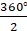⇒ x + y + z = 180° ------(1)

Given that, x : y : z = 2 : 3 : 5

Let x = 2m,y = 3m,z = 5m (∵ m = constant)

Substitute these values in equation (1) we get

2m + 3m + 5m = 180

10m = 180

m =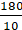∴ m = 18

Substituting m = 18 in x,y,z

x = 2m,x = 2(18) = 36°

y = 3m,y = 3(18) = 54°

z = 5m,z = 5(18) = 90°

∴ x = 36°,y = 54°,z = 90°

Question 2.

Find the value of x in the following figures.

i.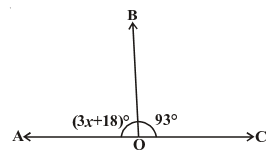ii.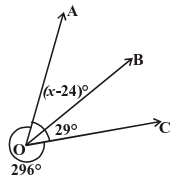iii.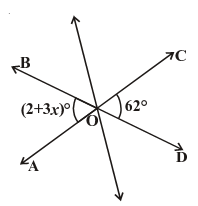iv.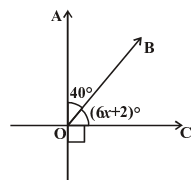(i) From the given figure,

3x + 18° + 93° = 180°

⇒ 3x + 111° = 180°

⇒ 3x = 180°-111°

⇒ 3x = 69°

⇒ x =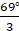∴ x = 24°

(ii) From the given figure,

(x-24)° + 29° + 296° = 360°

⇒ (x-24)° = 360°-325°

⇒ (x-24)° = 35°

⇒ x = 35° + 24°

∴ x = 59°

(iii) From the given figure,

(2 + 3x)° = 62°

⇒ 3x = 62°-2° = 60°

⇒ x =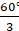∴ x = 20°

(iv) From the given figure,

40° + (6x + 2)° = 90°

⇒ (6x + 2)° = 90°-40°

⇒ (6x + 2)° = 50°

⇒ 6x = 50°-2° = 48°

⇒ x =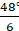∴ x = 8°

Question 3.

In the given figure lines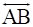and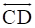intersect at O. If ∠AOC + ∠BOE = 70° and ∠BOD = 40°, find ∠BOE and reflex ∠COE.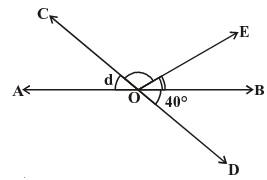Given that,

The linesand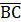intersect at O.

∠AOC + ∠BOE = 70° ----(1)

∠BOD = 40° ----(2)

If the two lines intersect at a point then its vertically opposite angles are equal.

∴ ∠AOC = ∠BOD

Substitute (2) in (1)

⇒ 40° + ∠BOE = 70°

⇒∠BOE = 70°-40°

∴∠BOE = 30°

From the figure,AOB is a straight line and its angle is 180°

So, ∠AOC + ∠BOE + ∠COE = 180°

From equation (1)

⇒ 70° + ∠COE = 180°

⇒∠COE = 180°-70°

∴∠COE = 110°

Reflex ∠COE = 360° - 110° = 250°

∴∠BOE = 30° and Reflex ∠COE = 250°

Question 4.

In the given figure lines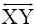and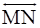intersect at O. If ∠POY = 90° and a: b = 2 : 3, find c.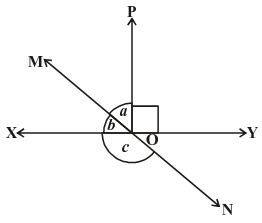Given that,

The lines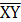and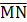intersect at O.

From the figure, XOY is a straight line and its angle is 180°

So, ∠XOM + ∠MOP + ∠POY = 180°

From the given, Let ∠a = 2x and ∠b = 3x

⇒∠b + ∠a + ∠POY = 180°----(1)

Given that ∠POY = 90°

Substitute the values in equation (1),

⇒2x + 3x + 90° = 180°

⇒5x + 90° = 180°

⇒5x = 180°-90° = 90°

⇒x =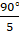∴ x = 18°

⇒ ∠a = 2x = 2×18° = 36°

⇒ ∠b = 3x = 3×18° = 54°

From the figure, MON is a straight line and its angle is 180°

⇒∠b + ∠c = 180°

⇒54° + ∠c = 180°

⇒∠c = 180°-54°

∴ ∠c = 126°

Question 5.

In the given figure ∠PQR = ∠PRQ, then prove that ∠PQS = ∠PRT.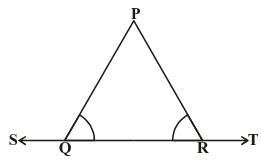In the figure, ST is a straight line and its angle is 180°

So, ∠PQS + ∠PQR = 180° ----(1)

And ∠PRT + ∠PRQ = 180°-----(2)

From the two equations, we get

∠PQS + ∠PQR = ∠PRT + ∠PRQ

Given that,

∠PQR = ∠PRQ

⇒ ∠PQS + ∠PRQ = ∠PRT + ∠PRQ

⇒ ∠PQS = ∠PRT + ∠PRQ -∠PRQ

⇒ ∠PQS = ∠PRT

So, ∠PQS = ∠PRT is proved.

Question 6.

In the given figure, if x + y = w + z, then prove that AOB is a line.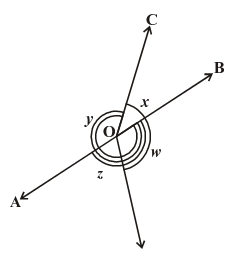In a circle, the sum of all angles is 360°

∴ ∠AOC + ∠BOC + ∠DOB + ∠AOD = 360°

⇒ x + y + w + z = 360°

Given that, x + y = w + z

⇒ w + z + w + z = 360°

⇒ 2w + 2z = 360°

⇒ 2(w + z) = 360°

⇒ w + z = 180° or ∠DOB + ∠AOD = 180°

If the sum of two adjacent angles is 180° then it forms a line.

So AOB is a line.

Question 7.

Given that,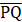is a line and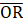is perpendicular to line.

⇒ ∠POR = 90°

The sum of linear pair is always equal to 180°

∴ ∠POS + ∠ROS + ∠POR = 180°

Substitute ∠POR = 90°

⇒90° + ∠POS + ∠ROS = 180°

⇒∠POS + ∠ROS = 90°

∴ ∠ROS = 90°-∠POS----(1)

⇒ ∠QOR = 90°

Given that OS is another ray lying between OP and OR

⇒∠QOS-∠ROS = 90°

∴∠ROS = ∠QOS-90°----(2)

On adding two equations (1) and (2) we get

2∠ROS = ∠QOS-∠POS

⇒ ∠ROS =(∠QOS-∠POS)

So, ∠ROS =(∠QOS-∠POS) is proved.

Question 8.

It is given that ∠XYZ = 64° and XY is produced to point P. A ray YQ bisects ∠ZYP. Draw a figure from the given information. Find ∠XYQ and reflex ∠QYP.

Let us draw a figure from the given,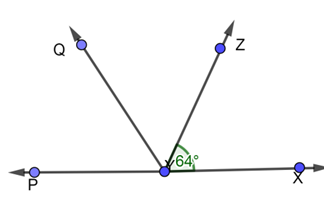Given that, a ray YQ bisects ∠ZYP

So, ∠QYP = ∠ZYQ

Here, PX is a straight line, so the sum of the angles is equal to 180°

∠XYZ + ∠ZYQ + ∠QYP = 180°

Given that, ∠XYZ = 64° and ∠QYP = ∠ZYQ

⇒ 64° + 2∠QYP = 180°

⇒ 2∠QYP = 180°-64° = 116°

∴ ∠QYP =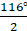= 58°

Also, ∠QYP = ∠ZYQ = 58°

Using the angle of reflection,

∠QYP = 360°-58° = 302°

∠XYQ = ∠XYZ + ∠ZYQ

⇒∠XYQ = 64° + 58° = 122°

∴ Reflex ∠QYP = 302° and ∠XYQ = 122°

## MATHS VIDEOS